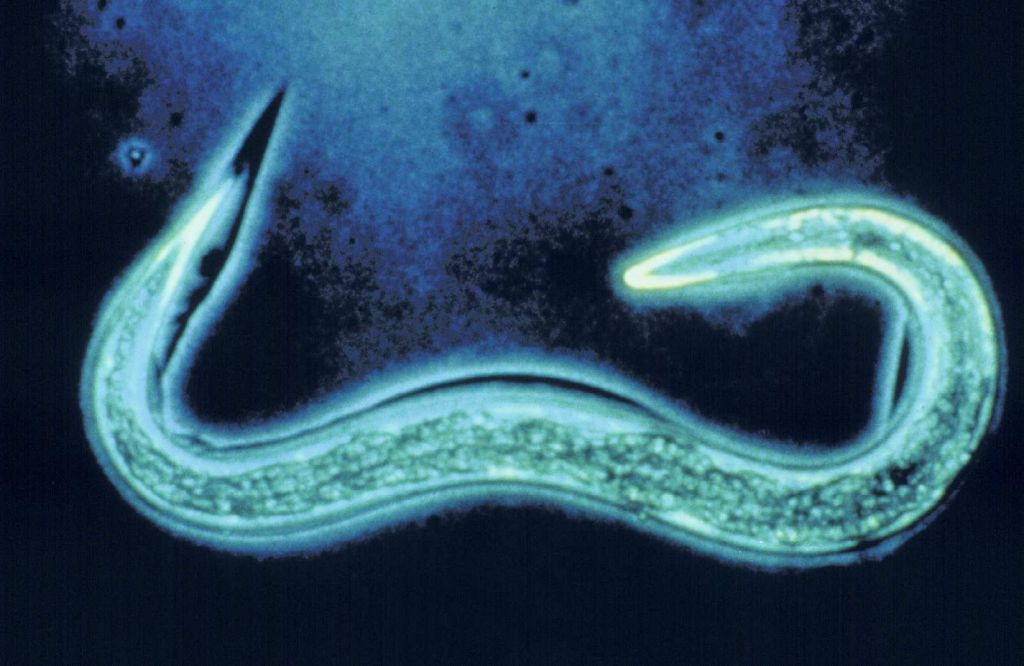The study of optimal swimming strategies at the microscopic scale draws interest from both fields of biological and robotic systems. In [2,3] we study the particular model of the so-called N-link swimmer, more precisely the 3-link swimmer introduced by Purcell in .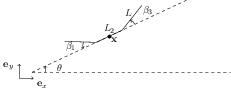Swimming at low Reynolds number, Resistive Force Theory
At the microscopic scale, the situation is the one of low Reynolds  numbers, with inertial forces neglected with respect to viscosity. Therefore the hydrodynamics of the system are governed by the Stokes equation, and the dynamics of the swimmer follow the Newton laws without inertia. Resistive Force Theory provides a local drag approximation, assuming that the force exerted on the swimmer by the fluid is linear with respect to velocity. In this framework, the dynamics of the N-link swimmer in a plane can be expressed as follows, noting$Latex formula$ the position and orientation of the swimmer, and$Latex formula$ the shape angles between two adjacent links:$Latex formula$

The dynamics has no drift term, meaning that without deformation of its shape, the swimmer remains motionless.

Optimal swimming problem
Noting the state$Latex formula$ and the control$Latex formula$, we can formulate the optimal swimming problem for different objective functions (maximal displacement, minimum time, minimum work, …).$Latex formula$

Simulations: maximal displacement along x-axis
We solve the above problem while maximizing horizontal displacement, with no a priori assumption on periodicity. We observe that the optimal trajectory is indeed a sequence of periodic strokes, different from the classical Purcell strokes. More precisely, the state constraints on the shape angles are active, the control structure has both bang and constrained arcs. The optimal stroke appears to be shorter than the classical Purcell stroke.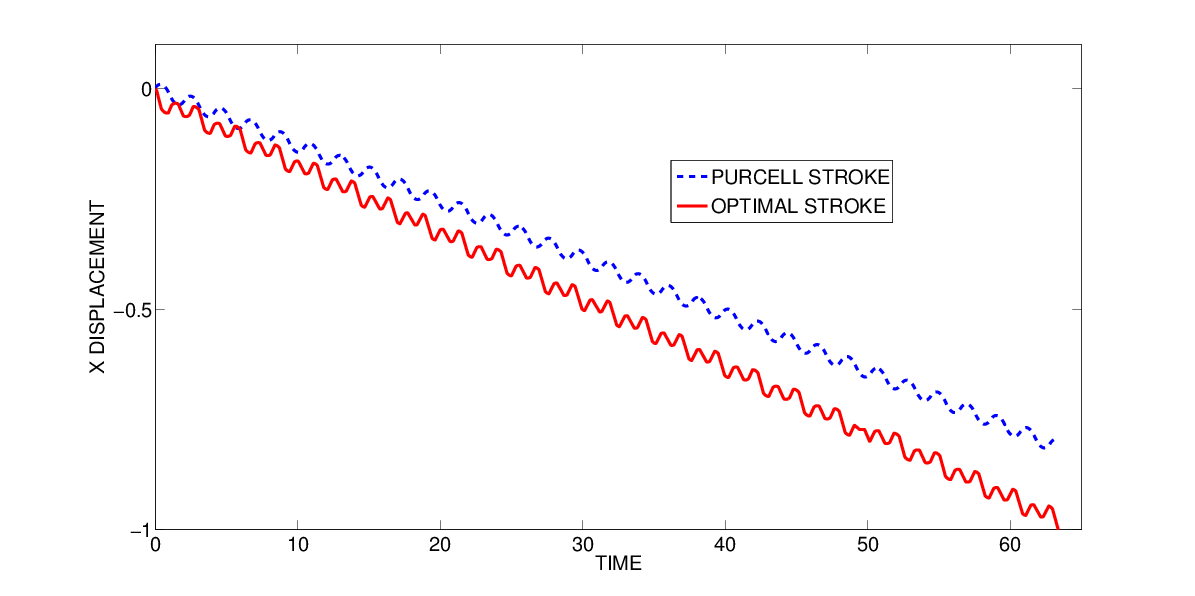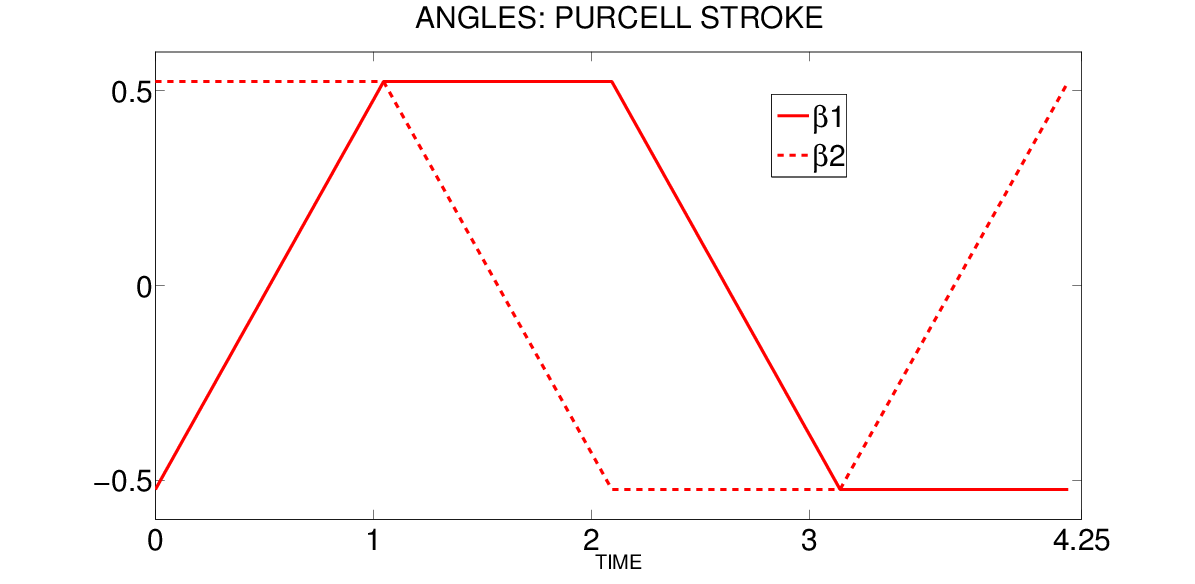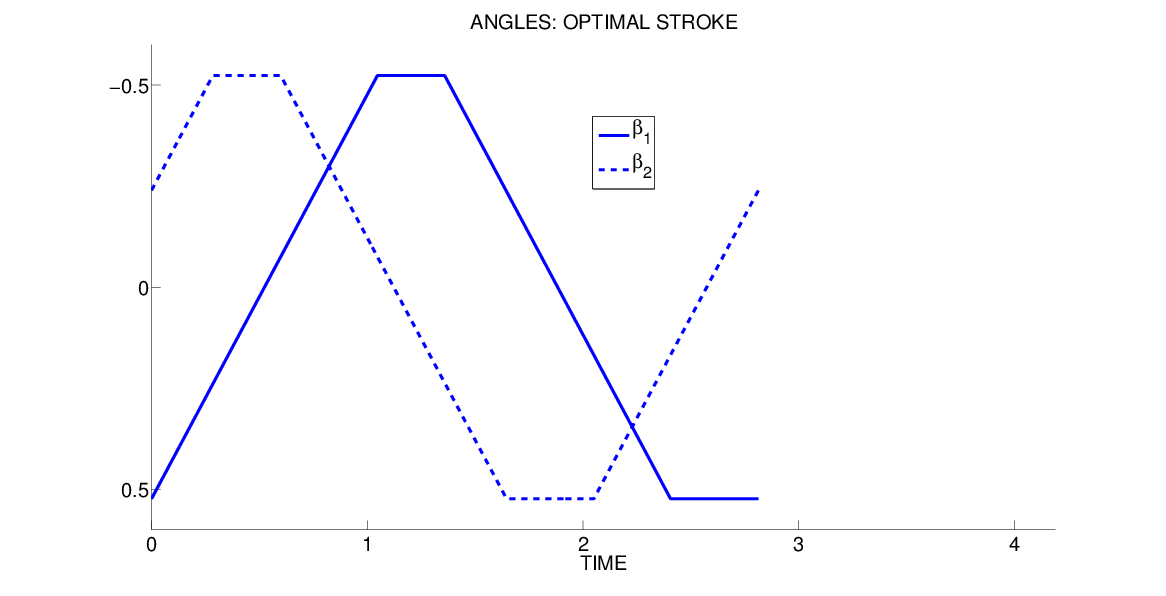Micro-swimmer optimal design
In  we address the question of the optimal length for each link, in order to maximize the displacement of the swimmer. The classical Purcell swimmer is defined by L_1=L_3=L=1, L_2=2, meaning the central link is twice as long as the other two. Assuming an octagonal-shaped stroke, we use an asymptotic expansion of the displacement for small amplitudes to derive the optimal ratio$Latex formula$

For a total length of 4 this corresponds to L*=1.4702, L_2*=1.0596.  Numerical simulations give results quite close to these values, and show a gain in displacement about 60%. We show below the comparison between the Purcell swimmer and the optimal swimmer for different deformation speed limits b, for an amplitude a=pi/6.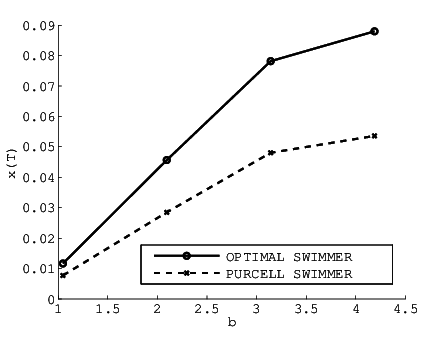Finally, if we set the speed limit to b=1 and solve the problem for  different amplitudes a, we observe a change in the stroke phase portrait. For large amplitudes, we obtain unconstrained solutions instead of  octagonal shapes. These solutions typically have a control structure with bang and singular arcs (instead of constrained arcs)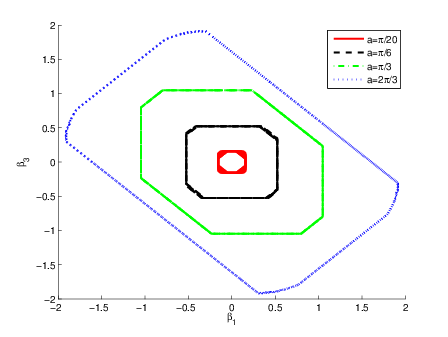Phase portrait for different amplitudes, including an unconstrained solution (blue)

References

 E. M. Purcell. Life at low reynolds number. American Journal of Physics, 45:3–11,1977.

 Laetitia Giraldi, Pierre Martinon, and Marta Zoppello. Controllability and Optimal Strokes for N-link Micro-swimmer. In 52nd IEEE Conference on Decision and Control, Firenze, Italy, 2013.

 Laetitia Giraldi, Pierre Martinon, and Marta Zoppello. Optimal   design of purcell’s three-link swimmer. Phys. Rev. E, 91:023012, 2015.